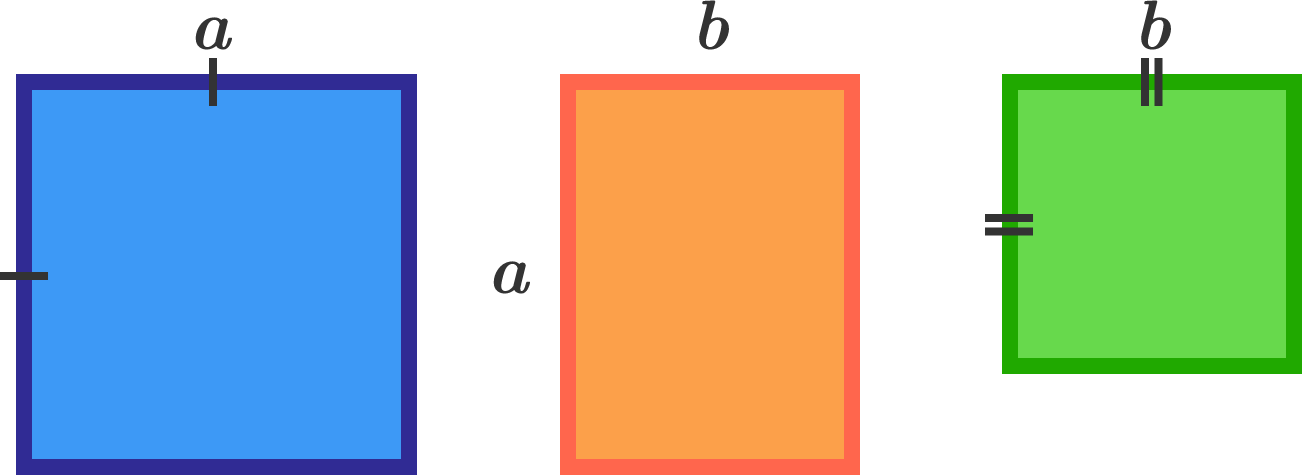# Block PartitionThree rectangles of dimensions $a\times a, a\times b,$ and $b\times b$ with $a$ and $b$ coprime integers are available as the bases on which to build cuboid towers of any heights. The tower on each base will be built with $1\times 1\times 1$ cubic blocks. For example, if we want to make cuboids of heights 1, 2, 3 for the first, second, and third bases, respectively, the total number of the unit blocks used will be $a^2+2ab+3b^2.$ But it is not required to use all three of the bases in building cuboid towers: you may use only one or two of the three bases.

You try all combinations of the bases and heights and find that, given 47 unit blocks, you can never build cuboid towers as described above, but that there is always a way to do the job if there are more than 47 blocks.

What is $a+b$?

×